# Missing Angles In A Quadrilateral Worksheet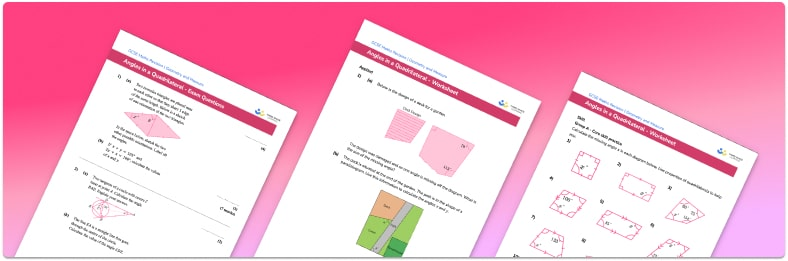• This field is for validation purposes and should be left unchanged.

You can unsubscribe at any time (each email we send will contain an easy way to unsubscribe). To find out more about how we use your data, see our privacy policy.

• Section 1 of the missing angles in a quadrilateral worksheet contains 36 skills-based missing angles in a quadrilateral questions, in 3 groups to support differentiation
• Section 2 contains 4 applied missing angles in a quadrilateral questions with a mix of worded problems and deeper problem solving questions
• Section 3 contains 4 foundation and higher level GCSE exam style questions
• Answers and a mark scheme for all questions are provided
• Questions follow variation theory with plenty of opportunities for students to work independently at their own level
• All questions created by fully qualified expert secondary maths teachers
• Suitable for GCSE maths revision for AQA, OCR and Edexcel exam boards
• Other printable angles worksheets for related topics, such as area of a parallelogram and area of compound shapes, are available.

### Missing angles in a quadrilateral at a glance

The interior angle sum in a quadrilateral is based on the axiom or postulate that (in standard Euclidean geometry) the sum of interior angles of a triangle is 180°. As any quadrilateral can be split into two triangles, the sum of the angles in any quadrilateral is 360°. This fact can be used to find an unknown angle in a quadrilateral, by summing the other angles and subtracting the total from 360°. This is useful when a diagram is not drawn to scale, so protractors or angle measures cannot be used.

Special quadrilaterals such as squares, rectangles and parallelograms have particular angle properties that should be used when solving these problems. For example, it is assumed that students will recognise right angles when marked at the vertices on a diagram, so these will not be given in figures. Similarly, they need to be able to recognise the markings for parallel lines. Opposite angles in a parallelogram are equal, as are base angles in an isosceles triangle, so these facts about known angles might be needed to solve some problems.

Angle problems don’t usually contain difficult fractions and decimals, but answers often need to be rounded to a given degree of accuracy.

Angles of a quadrilateral can be extended further to applications involving algebraic expressions and solving equations.

Looking forward, students can then progress to finding the size of angles in polygons, finding exterior angles, and using angle facts for parallel lines. There are also additional geometry practice worksheets available for a variety of other topics.For more teaching and learning support on Geometry our GCSE maths lessons provide step by step support for all GCSE maths concepts.

## Related worksheets

Area of a Circle Worksheet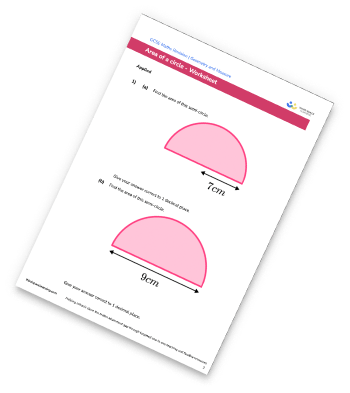Area of Compound Shapes Worksheet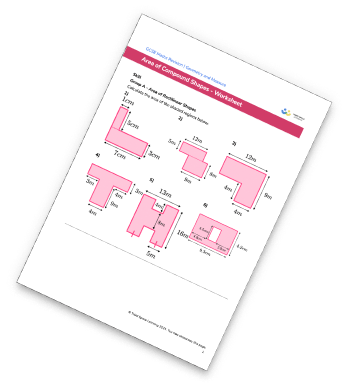Area Of A Trapezium Worksheet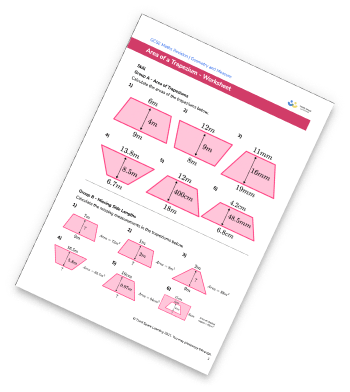Area Of A Rhombus Worksheet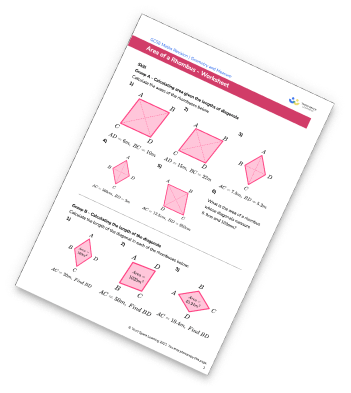## Do you have KS4 students who need more focused attention to succeed at GCSE?There will be students in your class who require individual attention to help them succeed in their maths GCSEs. In a class of 30, it’s not always easy to provide.

Help your students feel confident with exam-style questions and the strategies they’ll need to answer them correctly with our dedicated GCSE maths revision programme.

Lessons are selected to provide support where each student needs it most, and specially-trained GCSE maths tutors adapt the pitch and pace of each lesson. This ensures a personalised revision programme that raises grades and boosts confidence.

Find out more